International
Tables for
Crystallography
Volume B
Reciprocal space
Edited by U. Shmueli

International Tables for Crystallography (2006). Vol. B, ch. 5.1, pp. 548-550   | 1 | 2 |

Section 5.1.8. Real waves

A. Authiera*

aLaboratoire de Minéralogie-Cristallographie, Université P. et M. Curie, 4 Place Jussieu, F-75252 Paris CEDEX 05, France
Correspondence e-mail: authier@lmcp.jussieu.fr

5.1.8. Real waves

| top | pdf |

5.1.8.1. Introduction

| top | pdf |

The preceding sections have dealt with the diffraction of a plane wave by a semi-infinite perfect crystal. This situation is actually never encountered in practice, although with various devices, in particular using synchrotron radiation, it is possible to produce highly collimated monochromated waves which behave like pseudo plane waves. The wave from an X-ray tube is best represented by a spherical wave. The first experimental proof of this fact is due to Kato & Lang (1959)in the transmission case. Kato extended the dynamical theory to spherical waves for non-absorbing (Kato, 1961a,b) and absorbing crystals (Kato, 1968a,b). He expanded the incident spherical wave into plane waves by a Fourier transform, applied plane-wave dynamical theory to each of these components and took the Fourier transform of the result again in order to obtain the final solution. Another method for treating the problem was used by Takagi (1962, 1969), who solved the propagation equation in a medium where the lateral extension of the incident wave is limited and where the wave amplitudes depend on the lateral coordinates. He showed that in this case the set of fundamental linear equations (5.1.2.20)should be replaced by a set of partial differential equations. This treatment can be applied equally well to a perfect or to an imperfect crystal. In the case of a perfect crystal, Takagi showed that these equations have an analytical solution that is identical with Kato's result. Uragami (1969, 1970) observed the spherical wave in the Bragg (reflection) case, interpreting the observed intensity distribution using Takagi's theory. Saka et al. (1973) subsequently extended Kato's theory to the Bragg case.

Without using any mathematical treatment, it is possible to make some elementary remarks by considering the fact that the divergence of the incident beam falling on the crystal from the source is much larger than the angular width of the reflection domain. Fig. 5.1.8.1(a)shows a spatially collimated beam falling on a crystal in the transmission case and Fig. 5.1.8.1(b)represents the corresponding situation in reciprocal space. Since the divergence of the incident beam is larger than the angular width of the dispersion surface, the plane waves of its Fourier expansion will excite every point of both branches of the dispersion surface. The propagation directions of the corresponding wavefields will cover the angular range between those of the incident and reflected beams (Fig. 5.1.8.1a) and fill what is called the Borrmann triangle. The intensity distribution within this triangle has interesting properties, as described in the next two sections.Figure 5.1.8.1 | top | pdf |Borrmann triangle. When the incident beam is divergent, the whole dispersion surface is excited and the wavefields excited inside the crystal propagate within a triangle filling all the space between the incident direction, AC, and the reflected direction, AB. Along any direction Ap within this triangle two wavefields propagate, having as tie points two conjugate points, P and P′, at the extremities of a diameter of the dispersion surface. (a) Direct space; (b) reciprocal space.

5.1.8.2. Borrmann triangle

| top | pdf |

The first property of the Borrmann triangle is that the angular density of the wavefield paths is inversely proportional to the curvature of the dispersion surface around their tie points. Let us consider an incident wavepacket of angular width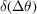. It will generate a packet of wavefields propagating within the Borrmann triangle. The angular width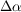(Fig. 5.1.8.2) between the paths of the corresponding wavefields is related to the radius of curvatureof the dispersion surface by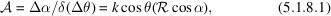where α is the angle between the wavefield path and the lattice planes [equation (5.1.2.26)] andis called the amplification ratio. In the middle of the reflecting domain, the radius of curvature of the dispersion surface is very much shorter than its value, k, far from it (about 104 times shorter) and the amplification ratio is therefore very large. As a consequence, the energy of a wavepacket of widthin reciprocal space is spread in direct space over an anglegiven by (5.1.8.1). The intensity distribution on the exit surface BC (Fig. 5.1.8.1a) is therefore proportional to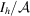. It is represented in Fig. 5.1.8.3for several values of the absorption coefficient:

• (i) Small values of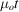(less than 2 or 3) (Fig. 5.1.8.3a). The intensity distribution presents a wide minimum in the centre where the density of wavefields is small and increases very sharply at the edges where the density of wavefields is large, although it is the reverse for the reflecting power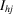. This effect, called the margin effect, was predicted qualitatively by Borrmann (1959)and von Laue (1960), demonstrated experimentally by Kato & Lang (1959), and calculated by Kato (1960).Figure 5.1.8.2 | top | pdf |Packet of wavefields of divergence Δα excited in the crystal by an incident wavepacket of angular width. (a) Direct space; (b) reciprocal space.Figure 5.1.8.3 | top | pdf |Intensity distribution along the base of the Borrmann triangle. y is a normalized coordinate along BC. (a) Small values of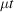. The interference (spherical-wave Pendellösung) between branch 1 and branch 2 is neglected. (b) Large values of.
• (ii) Large values of(of the order of 6 or more) (Fig. 5.1.8.3b). The predominant factor is now anomalous absorption. The wavefields propagating along the edges of the Borrmann triangle undergo normal absorption, while those propagating parallel to the lattice planes (or nearly parallel) correspond to tie points in the centre of the dispersion surface and undergo anomalously low absorption. The intensity distribution now has a maximum in the centre. For values oflarger than 10 or so, practically only the wavefields propagating parallel to the lattice planes go through the crystal, which acts as a wave guide: this is the Borrmann effect.

5.1.8.3. Spherical-wave Pendellösung

| top | pdf |

Fig. 5.1.8.4shows that along any path Ap inside the Borrmann triangle two wavefields propagate, one with tie point, on branch 1, the other with the point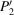, on branch 2. These two points lie on the extremities of a diameter of the dispersion surface. The two wavefields interfere, giving rise to Pendellösung fringes, which were first observed by Kato & Lang (1959), and calculated by Kato (1961b). These fringes are of course quite different from the plane-wave Pendellösung fringes predicted by Ewald (Section 5.1.6.3)because the tie points of the interfering wavefields are different and their period is also different, but they have in common the fact that they result from interference between wavefields belonging to different branches of the dispersion surface.Figure 5.1.8.4 | top | pdf |Interference at the origin of the Pendellösung fringes in the case of an incident spherical wave. (a) Direct space; (b) reciprocal space.

Kato has shown that the intensity distribution at any point at the base of the Borrmann triangle is proportional to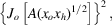where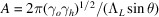and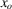and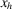are the distances of p from the sides AB and AC of the Borrmann triangle (Fig. 5.1.8.4). The equal-intensity fringes are therefore located along the locus of the points in the triangle for which the product of the distances to the sides is constant, that is hyperbolas having AB and AC as asymptotes (Fig. 5.1.8.4b). These fringes can be observed on a section topograph of a wedge-shaped crystal (Fig. 5.1.8.5). The technique of section topography is described in IT C, Section 2.7.2.2. The Pendellösung distance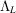depends on the polarization state [see equation (5.1.3.8)]. If the incident wave is unpolarized, one observes the superposition of the Pendellösung fringes corresponding to the two states of polarization, parallel and perpendicular to the plane of incidence. This results in a beat effect, which is clearly visible in Fig. 5.1.8.5.Figure 5.1.8.5 | top | pdf |Spherical-wave Pendellösung fringes observed on a wedge-shaped crystal. (a) Computer simulation (solid lines: maxima; dashed lines: minima). (b) X-ray section topograph of a wedge-shaped silicon crystal (444 reflection, Mo Kα radiation).

References

Borrmann, G. (1959). Röntgenwellenfelder. Beit. Phys. Chem. 20 Jahrhunderts, pp. 262–282. Braunschweig: Vieweg und Sohn.
Kato, N. (1960). The energy flow of X-rays in an ideally perfect crystal: comparison between theory and experiments. Acta Cryst. 13, 349–356.
Kato, N. (1961a). A theoretical study of Pendellösung fringes. Part I. General considerations. Acta Cryst. 14, 526–532.
Kato, N. (1961b). A theoretical study of Pendellösung fringes. Part 2. Detailed discussion based upon a spherical wave theory. Acta Cryst. 14, 627–636.
Kato, N. (1968a). Spherical-wave theory of dynamical X-ray diffraction for absorbing perfect crystals. I. The crystal wave fields. J. Appl. Phys. 39, 2225–2230.
Kato, N. (1968b). Spherical-wave theory of dynamical X-ray diffraction for absorbing perfect crystals. II. Integrated reflection power. J. Appl. Phys. 39, 2231–2237.
Kato, N. & Lang, A. R. (1959). A study of Pendellösung fringes in X-ray diffraction. Acta Cryst. 12, 787–794.
Laue, M. von (1960). Röntgenstrahl-Interferenzen. Frankfurt am Main: Akademische Verlagsgesellschaft.
Saka, T., Katagawa, T. & Kato, N. (1973). The theory of X-ray crystal diffraction for finite polyhedral crystals. III. The Bragg–(Bragg)m cases. Acta Cryst. A29, 192–200.
Takagi, S. (1962). Dynamical theory of diffraction applicable to crystals with any kind of small distortion. Acta Cryst. 15, 1311–1312.
Takagi, S. (1969). A dynamical theory of diffraction for a distorted crystal. J. Phys. Soc. Jpn, 26, 1239–1253.
Uragami, T. (1969). Pendellösung fringes of X-rays in Bragg case. J. Phys. Soc. Jpn, 27, 147–154.
Uragami, T. (1970). Pendellösung fringes in a crystal of finite thickness. J. Phys. Soc. Jpn, 28, 1508–1527.